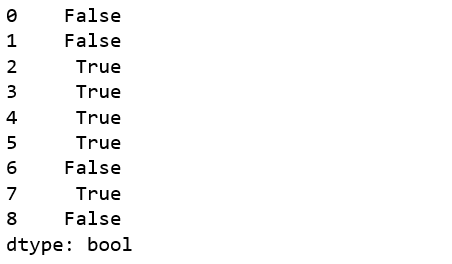# Python | Pandas Series.ge()

• Last Updated : 03 Jan, 2020

Python is a great language for doing data analysis, primarily because of the fantastic ecosystem of data-centric Python packages. Pandas is one of those packages and makes importing and analyzing data much easier.

Pandas` series.ge()` is used to compare every element of Caller series with passed series. It returns True for every element which is Greater than or Equal to the element in passed series.

Note: The results are returned on the basis of comparison caller series >= other series.

Syntax: Series.ge(other, level=None, fill_value=None, axis=0)

Parameters:
other: other series to be compared with
level: int or name of level in case of multi level
fill_value: Value to be replaced instead of NaN
axis: 0 or ‘index’ to apply method by rows and 1 or ‘columns’ to apply by columns.

Return type: Boolean series

Example #1: NaN handling

In this example, two series are created using `pd.Series()`. The series contains some Null values and some equal values at same indices too. The series are compared using `.ge() `method and 7 is passed to fill_value parameter to replace NaN values by 7.

 `# importing pandas module  ``import` `pandas as pd  ``   ` `# importing numpy module ``import` `numpy as np ``   ` `# creating series 1 ``series1 ``=` `pd.Series([``70``, ``0``, ``2``, ``225``, ``1``, ``16``, np.nan, ``10``, np.nan]) ``   ` `# creating series 2 ``series2 ``=` `pd.Series([``27``, np.nan, ``2``, ``23``, ``5``, ``95``, ``4``, ``3``, ``19``]) `` ` `# NaN replacement``replace_nan ``=` `7`` ` `# calling and returning to result variable``result ``=` `series1.ge(series2, fill_value ``=` `replace_nan)`` ` `# display ``result `

Output:
As shown in output, True was returned wherever value in caller series was Greater than or Equal value in passed series. Also it can be seen Null values were replaced by 7 and the comparison was made using that value.Example #2: Calling on Series with str objects

In this example, two series are created using `pd.Series()`. The series contains some string values too. In case of strings, the comparison is made with their ASCII values.

 `# importing pandas module  ``import` `pandas as pd  ``   ` `# importing numpy module ``import` `numpy as np ``   ` `# creating series 1 ``series1 ``=` `pd.Series([``'A'``, ``0``, ``'c'``, ``43``, ``9``, ``'e'``, np.nan, ``'x'``, np.nan]) ``   ` `# creating series 2 ``series2 ``=` `pd.Series([``'v'``, np.nan, ``'c'``, ``23``, ``5``, ``'D'``, ``54``, ``'p'``, ``19``]) `` ` `# NaN replacement``replace_nan ``=` `14`` ` `# calling and returning to result variable``result ``=` `series1.ge(series2, fill_value ``=` `replace_nan)`` ` `# display ``result `

Output:
As it can be seen in output, in case of strings, the comparison was made using their ASCII values.My Personal Notes arrow_drop_up